# bcs_unit-6.ppt

2 de Jun de 2023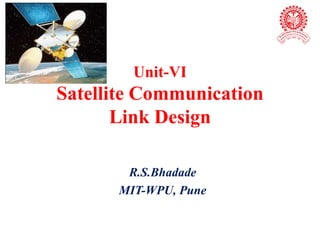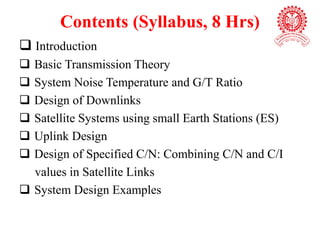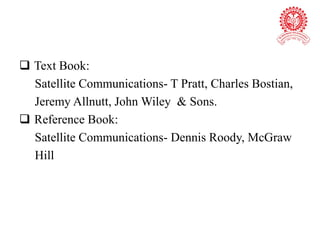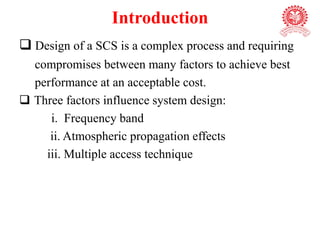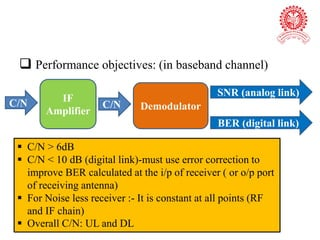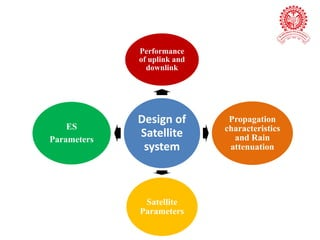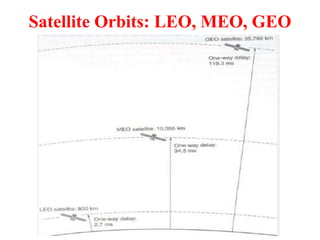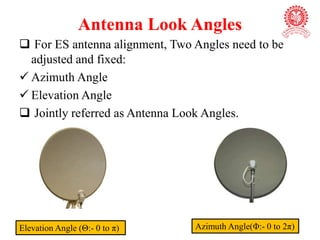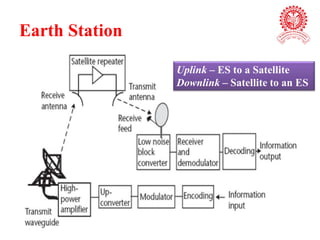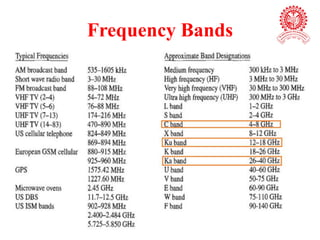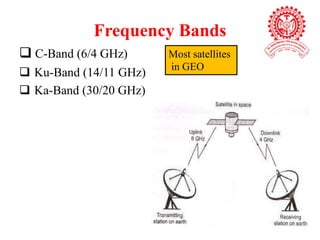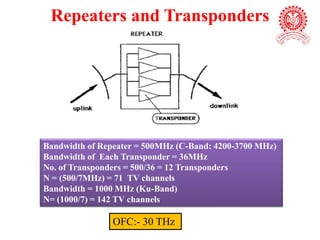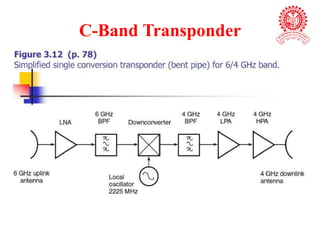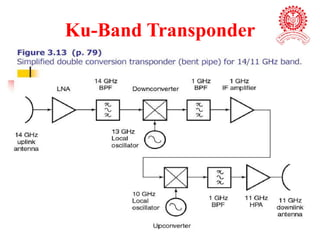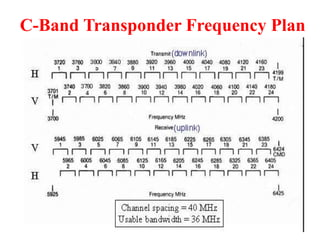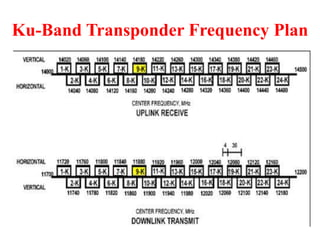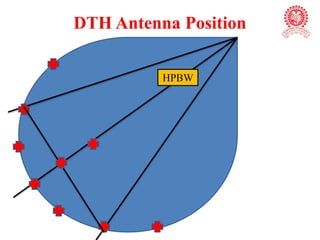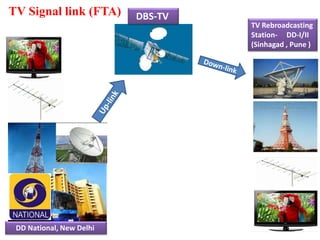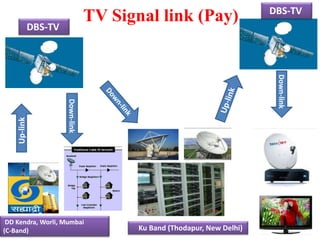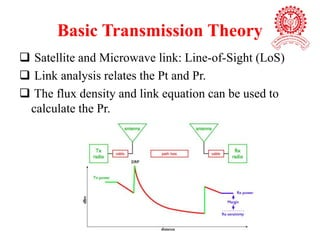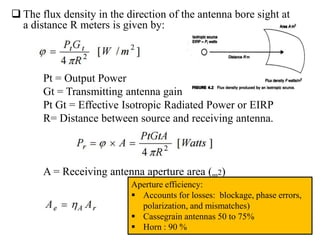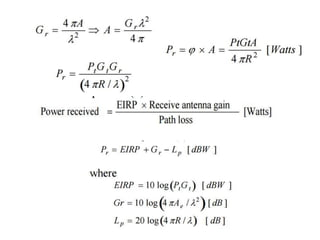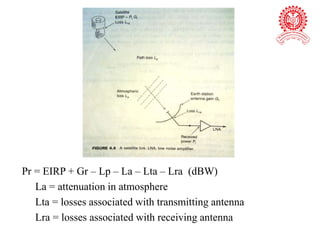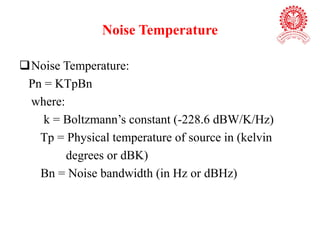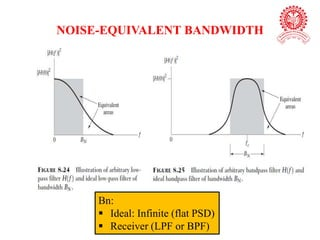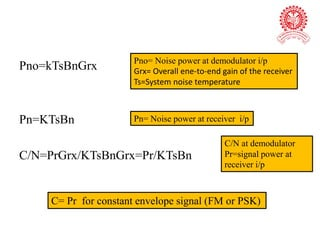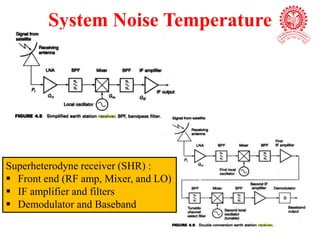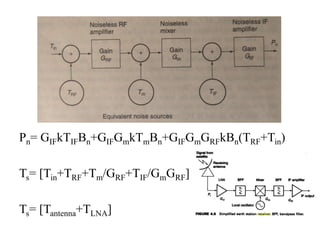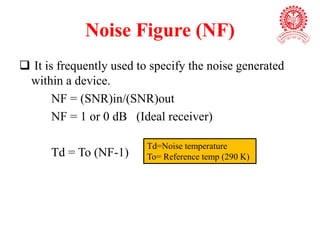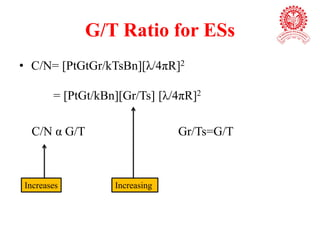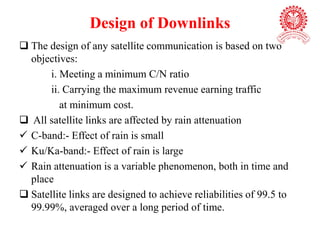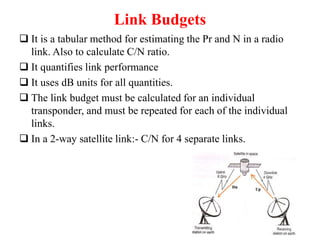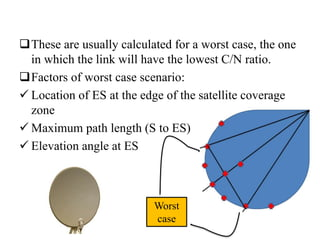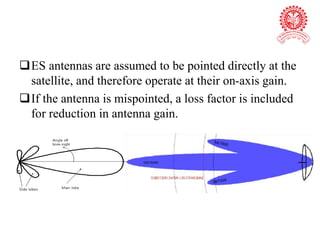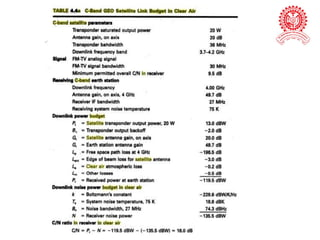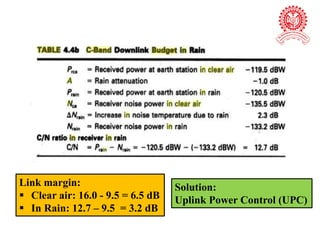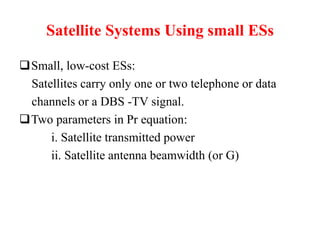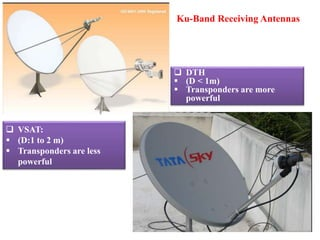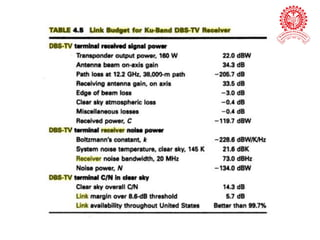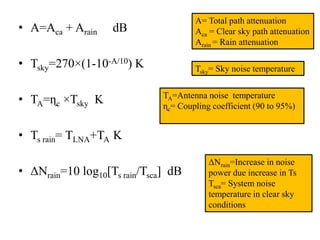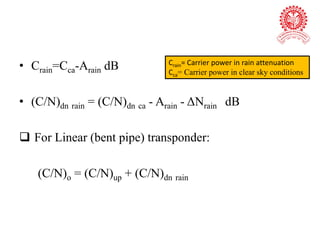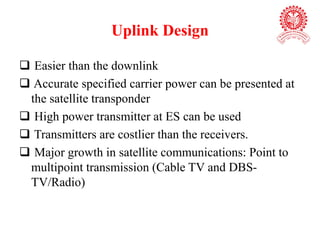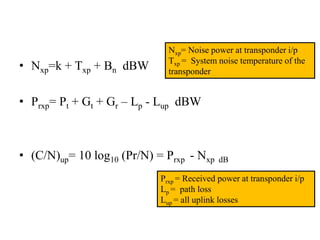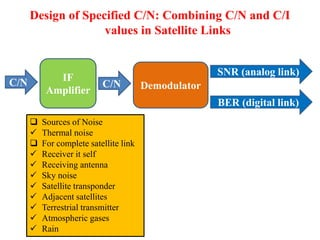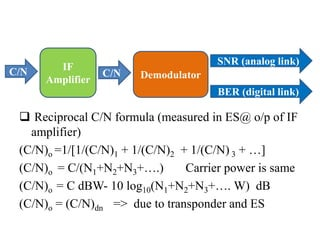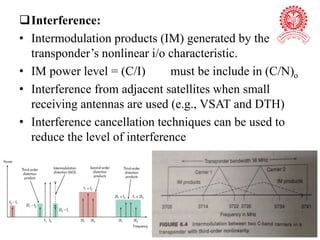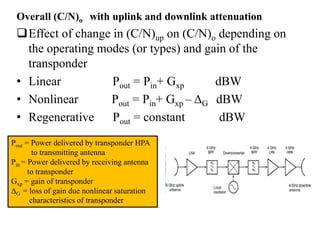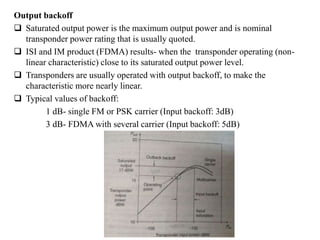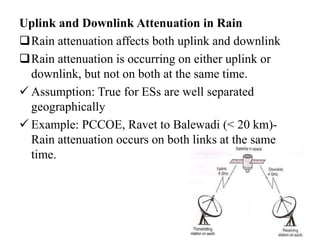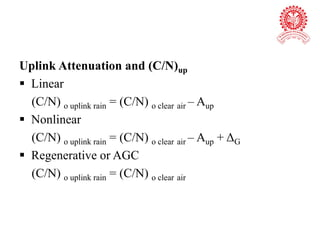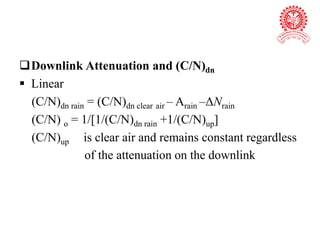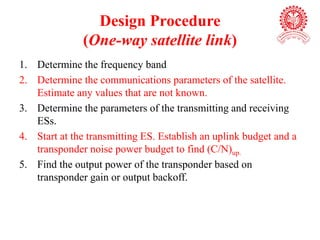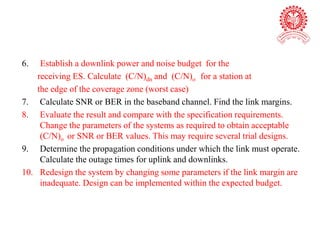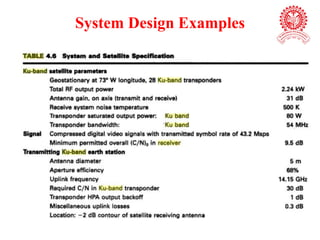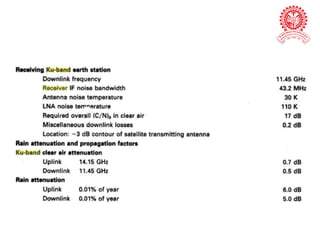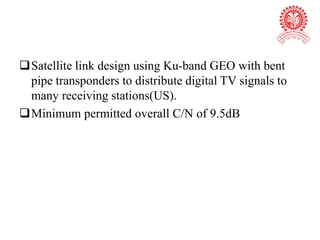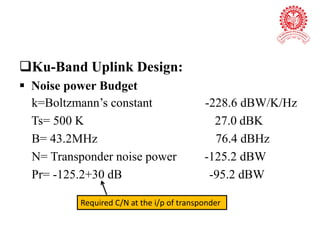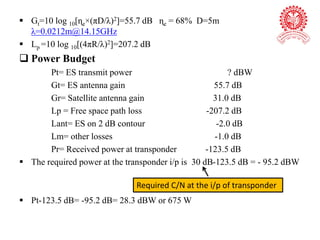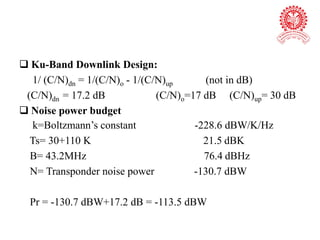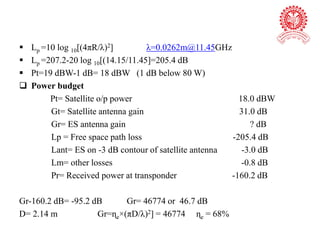1 de 62

### bcs_unit-6.ppt

• 2. Contents (Syllabus, 8 Hrs)  Introduction  Basic Transmission Theory  System Noise Temperature and G/T Ratio  Design of Downlinks  Satellite Systems using small Earth Stations (ES)  Uplink Design  Design of Specified C/N: Combining C/N and C/I values in Satellite Links  System Design Examples
• 3.  Text Book: Satellite Communications- T Pratt, Charles Bostian, Jeremy Allnutt, John Wiley & Sons.  Reference Book: Satellite Communications- Dennis Roody, McGraw Hill
• 4. Introduction  Design of a SCS is a complex process and requiring compromises between many factors to achieve best performance at an acceptable cost.  Three factors influence system design: i. Frequency band ii. Atmospheric propagation effects iii. Multiple access technique
• 5.  Performance objectives: (in baseband channel) Demodulator C/N BER (digital link) SNR (analog link)  C/N > 6dB  C/N < 10 dB (digital link)-must use error correction to improve BER calculated at the i/p of receiver ( or o/p port of receiving antenna)  For Noise less receiver :- It is constant at all points (RF and IF chain)  Overall C/N: UL and DL IF Amplifier C/N
• 6. Design of Satellite system Performance of uplink and downlink Propagation characteristics and Rain attenuation Satellite Parameters ES Parameters
• 8. Antenna Look Angles  For ES antenna alignment, Two Angles need to be adjusted and fixed:  Azimuth Angle  Elevation Angle  Jointly referred as Antenna Look Angles. Elevation Angle (Θ:- 0 to π) Azimuth Angle(Φ:- 0 to 2π)
• 9. Earth Station Uplink – ES to a Satellite Downlink – Satellite to an ES
• 10. Satellite Functions (RF to RF conversion) 1. Signal reception: Uplink frequency 2. Processing of signal: Amplification, Filtration, and Down conversion, etc 3. Signal retransmission: Downlink frequency
• 12. Frequency Bands  C-Band (6/4 GHz)  Ku-Band (14/11 GHz)  Ka-Band (30/20 GHz) Most satellites in GEO
• 13. Repeaters and Transponders Bandwidth of Repeater = 500MHz (C-Band: 4200-3700 MHz) Bandwidth of Each Transponder = 36MHz No. of Transponders = 500/36 = 12 Transponders N = (500/7MHz) = 71 TV channels Bandwidth = 1000 MHz (Ku-Band) N= (1000/7) = 142 TV channels OFC:- 30 THz
• 19. TV Signal link (FTA) TV Rebroadcasting Station- DD-I/II (Sinhagad , Pune ) DD National, New Delhi DBS-TV
• 21. Basic Transmission Theory  Satellite and Microwave link: Line-of-Sight (LoS)  Link analysis relates the Pt and Pr.  The flux density and link equation can be used to calculate the Pr.
• 22.  The flux density in the direction of the antenna bore sight at a distance R meters is given by: Pt = Output Power Gt = Transmitting antenna gain Pt Gt = Effective Isotropic Radiated Power or EIRP R= Distance between source and receiving antenna. A = Receiving antenna aperture area (m2) Aperture efficiency:  Accounts for losses: blockage, phase errors, polarization, and mismatches)  Cassegrain antennas 50 to 75%  Horn : 90 %
• 24. Pr = EIRP + Gr – Lp – La – Lta – Lra (dBW) La = attenuation in atmosphere Lta = losses associated with transmitting antenna Lra = losses associated with receiving antenna
• 25. Noise Temperature Noise Temperature: Pn = KTpBn where: k = Boltzmann’s constant (-228.6 dBW/K/Hz) Tp = Physical temperature of source in (kelvin degrees or dBK) Bn = Noise bandwidth (in Hz or dBHz)
• 26. NOISE-EQUIVALENT BANDWIDTH Bn:  Ideal: Infinite (flat PSD)  Receiver (LPF or BPF)
• 27. Pno=kTsBnGrx Pn=KTsBn C/N=PrGrx/KTsBnGrx=Pr/KTsBn Pno= Noise power at demodulator i/p Grx= Overall ene-to-end gain of the receiver Ts=System noise temperature Pn= Noise power at receiver i/p C/N at demodulator Pr=signal power at receiver i/p C= Pr for constant envelope signal (FM or PSK)
• 28. System Noise Temperature Superheterodyne receiver (SHR) :  Front end (RF amp, Mixer, and LO)  IF amplifier and filters  Demodulator and Baseband
• 30. Noise Figure (NF)  It is frequently used to specify the noise generated within a device. NF = (SNR)in/(SNR)out NF = 1 or 0 dB (Ideal receiver) Td = To (NF-1) Td=Noise temperature To= Reference temp (290 K)
• 31. G/T Ratio for ESs • C/N= [PtGtGr/kTsBn][λ/4πR]2 = [PtGt/kBn][Gr/Ts] [λ/4πR]2 C/N α G/T Gr/Ts=G/T Increasing Increases
• 32. Design of Downlinks  The design of any satellite communication is based on two objectives: i. Meeting a minimum C/N ratio ii. Carrying the maximum revenue earning traffic at minimum cost.  All satellite links are affected by rain attenuation  C-band:- Effect of rain is small  Ku/Ka-band:- Effect of rain is large  Rain attenuation is a variable phenomenon, both in time and place  Satellite links are designed to achieve reliabilities of 99.5 to 99.99%, averaged over a long period of time.
• 33. Link Budgets  It is a tabular method for estimating the Pr and N in a radio link. Also to calculate C/N ratio.  It quantifies link performance  It uses dB units for all quantities.  The link budget must be calculated for an individual transponder, and must be repeated for each of the individual links.  In a 2-way satellite link:- C/N for 4 separate links.
• 34. These are usually calculated for a worst case, the one in which the link will have the lowest C/N ratio. Factors of worst case scenario:  Location of ES at the edge of the satellite coverage zone  Maximum path length (S to ES)  Elevation angle at ES Worst case
• 35. ES antennas are assumed to be pointed directly at the satellite, and therefore operate at their on-axis gain. If the antenna is mispointed, a loss factor is included for reduction in antenna gain.
• 37. Link margin:  Clear air: 16.0 - 9.5 = 6.5 dB  In Rain: 12.7 – 9.5 = 3.2 dB Solution: Uplink Power Control (UPC)
• 38. Satellite Systems Using small ESs Small, low-cost ESs: Satellites carry only one or two telephone or data channels or a DBS -TV signal. Two parameters in Pr equation: i. Satellite transmitted power ii. Satellite antenna beamwidth (or G)
• 39. Ku-Band Receiving Antennas  DTH  (D < 1m)  Transponders are more powerful  VSAT:  (D:1 to 2 m)  Transponders are less powerful
• 41. • A=Aca + Arain dB • Tsky=270×(1-10-A/10) K • TA=ɳc ×Tsky K • Ts rain= TLNA+TA K • ΔNrain=10 log10[Ts rain/Tsca] dB A= Total path attenuation Aca = Clear sky path attenuation Arain = Rain attenuation Tsky= Sky noise temperature TA=Antenna noise temperature ɳc= Coupling coefficient (90 to 95%) ΔNrain=Increase in noise power due increase in Ts Tsca= System noise temperature in clear sky conditions
• 42. • Crain=Cca-Arain dB • (C/N)dn rain = (C/N)dn ca - Arain - ΔNrain dB  For Linear (bent pipe) transponder: (C/N)o = (C/N)up + (C/N)dn rain Crain= Carrier power in rain attenuation Cca= Carrier power in clear sky conditions
• 43. Uplink Design  Easier than the downlink  Accurate specified carrier power can be presented at the satellite transponder  High power transmitter at ES can be used  Transmitters are costlier than the receivers.  Major growth in satellite communications: Point to multipoint transmission (Cable TV and DBS- TV/Radio)
• 44. • Nxp=k + Txp + Bn dBW • Prxp= Pt + Gt + Gr – Lp - Lup dBW • (C/N)up= 10 log10 (Pr/N) = Prxp - Nxp dB Nxp= Noise power at transponder i/p Txp = System noise temperature of the transponder Prxp = Received power at transponder i/p Lp = path loss Lup = all uplink losses
• 45. Design of Specified C/N: Combining C/N and C/I values in Satellite Links  Sources of Noise  Thermal noise  For complete satellite link  Receiver it self  Receiving antenna  Sky noise  Satellite transponder  Adjacent satellites  Terrestrial transmitter  Atmospheric gases  Rain Demodulator C/N BER (digital link) SNR (analog link) IF Amplifier C/N
• 46.  Reciprocal C/N formula (measured in ES@ o/p of IF amplifier) (C/N)o =1/[1/(C/N)1 + 1/(C/N)2 + 1/(C/N) 3 + …] (C/N)o = C/(N1+N2+N3+….) Carrier power is same (C/N)o = C dBW- 10 log10(N1+N2+N3+…. W) dB (C/N)o = (C/N)dn => due to transponder and ES Demodulator C/N BER (digital link) SNR (analog link) IF Amplifier C/N
• 47. Interference: • Intermodulation products (IM) generated by the transponder’s nonlinear i/o characteristic. • IM power level = (C/I) must be include in (C/N)o • Interference from adjacent satellites when small receiving antennas are used (e.g., VSAT and DTH) • Interference cancellation techniques can be used to reduce the level of interference
• 48. Overall (C/N)o with uplink and downlink attenuation Effect of change in (C/N)up on (C/N)o depending on the operating modes (or types) and gain of the transponder • Linear Pout = Pin+ Gxp dBW • Nonlinear Pout = Pin+ Gxp – ΔG dBW • Regenerative Pout = constant dBW Pout = Power delivered by transponder HPA to transmitting antenna Pin = Power delivered by receiving antenna to transponder Gxp = gain of transponder ΔG = loss of gain due nonlinear saturation characteristics of transponder
• 49. Output backoff  Saturated output power is the maximum output power and is nominal transponder power rating that is usually quoted.  ISI and IM product (FDMA) results- when the transponder operating (non- linear characteristic) close to its saturated output power level.  Transponders are usually operated with output backoff, to make the characteristic more nearly linear.  Typical values of backoff: 1 dB- single FM or PSK carrier (Input backoff: 3dB) 3 dB- FDMA with several carrier (Input backoff: 5dB)
• 50. Uplink and Downlink Attenuation in Rain Rain attenuation affects both uplink and downlink Rain attenuation is occurring on either uplink or downlink, but not on both at the same time.  Assumption: True for ESs are well separated geographically  Example: PCCOE, Ravet to Balewadi (< 20 km)- Rain attenuation occurs on both links at the same time.
• 51. Uplink Attenuation and (C/N)up  Linear (C/N) o uplink rain = (C/N) o clear air – Aup  Nonlinear (C/N) o uplink rain = (C/N) o clear air – Aup + ΔG  Regenerative or AGC (C/N) o uplink rain = (C/N) o clear air
• 52. Downlink Attenuation and (C/N)dn  Linear (C/N)dn rain = (C/N)dn clear air – Arain –ΔNrain (C/N) o = 1/[1/(C/N)dn rain +1/(C/N)up] (C/N)up is clear air and remains constant regardless of the attenuation on the downlink
• 53. Design Procedure (One-way satellite link) 1. Determine the frequency band 2. Determine the communications parameters of the satellite. Estimate any values that are not known. 3. Determine the parameters of the transmitting and receiving ESs. 4. Start at the transmitting ES. Establish an uplink budget and a transponder noise power budget to find (C/N)up. 5. Find the output power of the transponder based on transponder gain or output backoff.
• 54. 6. Establish a downlink power and noise budget for the receiving ES. Calculate (C/N)dn and (C/N)o for a station at the edge of the coverage zone (worst case) 7. Calculate SNR or BER in the baseband channel. Find the link margins. 8. Evaluate the result and compare with the specification requirements. Change the parameters of the systems as required to obtain acceptable (C/N)o or SNR or BER values. This may require several trial designs. 9. Determine the propagation conditions under which the link must operate. Calculate the outage times for uplink and downlinks. 10. Redesign the system by changing some parameters if the link margin are inadequate. Design can be implemented within the expected budget.
• 57. Satellite link design using Ku-band GEO with bent pipe transponders to distribute digital TV signals to many receiving stations(US). Minimum permitted overall C/N of 9.5dB
• 58. Ku-Band Uplink Design:  Noise power Budget k=Boltzmann’s constant -228.6 dBW/K/Hz Ts= 500 K 27.0 dBK B= 43.2MHz 76.4 dBHz N= Transponder noise power -125.2 dBW Pr= -125.2+30 dB -95.2 dBW Required C/N at the i/p of transponder
• 59.  Gt=10 log 10[ɳe×(πD/λ)2]=55.7 dB ɳe = 68% D=5m λ=0.0212m@14.15GHz  Lp =10 log 10[(4πR/λ)2]=207.2 dB  Power Budget Pt= ES transmit power ? dBW Gt= ES antenna gain 55.7 dB Gr= Satellite antenna gain 31.0 dB Lp = Free space path loss -207.2 dB Lant= ES on 2 dB contour -2.0 dB Lm= other losses -1.0 dB Pr= Received power at transponder -123.5 dB  The required power at the transponder i/p is 30 dB-123.5 dB = - 95.2 dBW  Pt-123.5 dB= -95.2 dB= 28.3 dBW or 675 W Required C/N at the i/p of transponder
• 60.  Ku-Band Downlink Design: 1/ (C/N)dn = 1/(C/N)o - 1/(C/N)up (not in dB) (C/N)dn = 17.2 dB (C/N)o=17 dB (C/N)up= 30 dB  Noise power budget k=Boltzmann’s constant -228.6 dBW/K/Hz Ts= 30+110 K 21.5 dBK B= 43.2MHz 76.4 dBHz N= Transponder noise power -130.7 dBW Pr = -130.7 dBW+17.2 dB = -113.5 dBW
• 61.  Lp =10 log 10[(4πR/λ)2] λ=0.0262m@11.45GHz  Lp =207.2-20 log 10[(14.15/11.45]=205.4 dB  Pt=19 dBW-1 dB= 18 dBW (1 dB below 80 W)  Power budget Pt= Satellite o/p power 18.0 dBW Gt= Satellite antenna gain 31.0 dB Gr= ES antenna gain ? dB Lp = Free space path loss -205.4 dB Lant= ES on -3 dB contour of satellite antenna -3.0 dB Lm= other losses -0.8 dB Pr= Received power at transponder -160.2 dB Gr-160.2 dB= -95.2 dB Gr= 46774 or 46.7 dB D= 2.14 m Gr=ɳe×(πD/λ)2] = 46774 ɳe = 68%